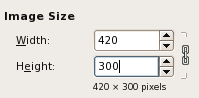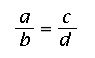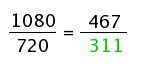# Calculating Image Aspect RatiosEver wonder how programs like Photoshop or The GIMP calculate image aspect ratios? No? Me neither. But, I was recently tasked with crunching such numbers, and I found the answer to be quite interesting. One thing I really hate is when somebody has incorrectly specified the `height` and `width` properties of an image. It’s always too tall or too wide, and looks quite atrocious. In the past, I’ve just opened up The GIMP real quick, created a blank image the size of my original, and then used the Scale Image function to see my new dimensions. Now, thanks to my newly-acquired knowledge, I won’t have to go through all that rigamarole.

Basically, it’s a simple math formula:

The product of the means is equal to the product of the extremes.Where b and c are the means, and a and d are the extremes.

So (b * c) / a = d, or(768 * 800) / 1024 = 600

In the example above, all the black numbers are known. You know the dimensions of your original image (1024x768), and you know your desired width (800). We only need to calculate the desired height (600). This simple formula works with basic 4:3 ratios like the example above, but also works on any ratio you give it:(720 * 467) / 1080 = 311
(with a little rounding)

I haven’t explained the formula in its original algebraic format, simply because it makes more sense to me this way. Sorry, math purists!

So, even though I can do these ratios with just a calculator, a friend (who wishes to remain anonymous) and I created a simple REBOL function and then a GUI version that does all the work for you. You can download those here (Right-click, “Save As…” to download the scripts):
If you use and/or like these scripts, let me know; I always like feedback.